#### Backward search

Backward versions of any of the forward search algorithms of Section 2.2.2 can be made. For example, a backward version of Dijkstra's algorithm can be made by starting from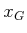. To create backward search algorithms, suppose that there is a single goal state,. For many planning problems, it might be the case that the branching factor is large when starting from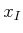. In this case, it might be more efficient to start the search at a goal state and work backward until the initial state is encountered. A general template for this approach is given in Figure 2.6. For forward search, recall that an action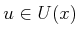is applied from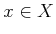to obtain a new state,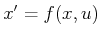. For backward search, a frequent computation will be to determine for some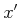, the preceding state, and actionsuch that. The template in Figure 2.6 can be extended to handle a goal region,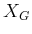, by inserting all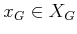into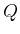in line 1 and marking them as visited.

For most problems, it may be preferable to precompute a representation of the state transition function,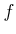, that is backward'' to be consistent with the search algorithm. Some convenient notation will now be constructed for the backward version of. Let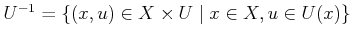, which represents the set of all state-action pairs and can also be considered as the domain of. Imagine from a given state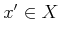, the set of all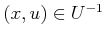that map tousing. This can be considered as a backward action space, defined formally for anyas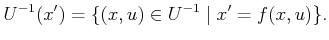(2.3)

For convenience, let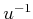denote a state-action pair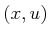that belongs to some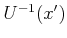. From any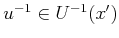, there is a unique. Thus, let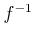denote a backward state transition function that yieldsfromand. This defines a backward state transition equation,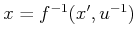, which looks very similar to the forward version,.

The interpretation ofis easy to capture in terms of the state transition graph: reverse the direction of every edge. This makes finding a plan in the reversed graph using backward search equivalent to finding one in the original graph using forward search. The backward state transition function is the variant ofthat is obtained after reversing all of the edges. Eachis a reversed edge. Since there is a perfect symmetry with respect to the forward search of Section 2.2.1, any of the search algorithm variants from Section 2.2.2 can be adapted to the template in Figure 2.6, provided thathas been defined.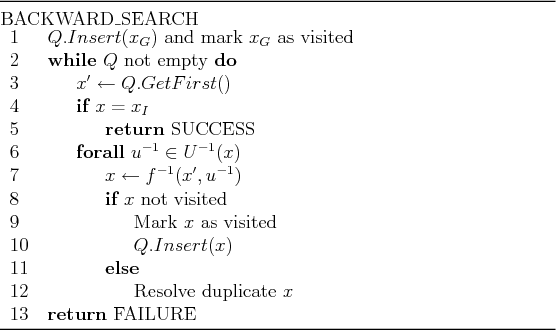Steven M LaValle 2020-08-14